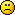This is a read-only archive of the old Scratch 1.x Forums.
Try searching the current Scratch discussion forums.

## #1 2013-04-30 12:00:04

harleycurnow
Scratcher
Registered: 2009-01-09
Posts: 24

### Realistic collisions

How do I make realistic collisions for a football(soccer) game? when the ball hits a sprite i want it to bounce off at a realistic angle. The players will be round counters and i've tried things like turning the ball 180 degrees but that only works if the ball hits dead centre of the player. I'm intermediate/advanced and this has baffled me. Is it even possible? PLEASE HELPOffline

## #2 2013-04-30 12:44:19

firedrake969_test
Scratcher
Registered: 2012-08-08
Posts: 500+

### Re: Realistic collisions

I'm pretty sure it uses trig.... let me think.  Is trig fine?

Alt of Firedrake969.

Offline

## #3 2013-04-30 12:50:04

harleycurnow
Scratcher
Registered: 2009-01-09
Posts: 24

### Re: Realistic collisions

Cant see how trig would come into it but now you mention it I have thought of a way it may be possible. I'll go test it and upload the results.

Offline

## #4 2013-05-03 11:32:40

cauzality
New Scratcher
Registered: 2013-03-19
Posts: 44

### Re: Realistic collisions

you will need some intermediate/advanced math but you don't need trig. do you know what vectors are? You will need to know how to project vectors onto other vectors. If you don't, just say so and ill try to explain/provide links about them. The rest of this post will assume that you do and will explain what you need to do to make the ball bounce.

It's easiest if it bounces off of something round, like another ball. You will need two vectors to start with: the velocity of the ball and the difference in positions of the ball and the thing it's bouncing off of. The difference vector has an x-component which is just the ball's x position minus the other thing's x position. It's y-component is the ball's y position minus the other thing's y position. Imagine an arrow pointing from the center of the other thing to the center of the ball.

You will use these two vectors to calculate a third: the projection of the velocity onto the difference vector. So think of the velocity vector as having two components, kind of like x and y, only now its components are the projection or the part that points parallel to the difference vector and another component which points in a perpendicular direction.

To reflect the ball, you change the sign of the component of the velocity vector which is parallel to the difference vector. You can do this by subtracting twice the parallel component from the original velocity vector (just like you can change 1 to -1 by subtracting 2).

Offline

## #5 2013-05-03 12:43:21

harleycurnow
Scratcher
Registered: 2009-01-09
Posts: 24

### Re: Realistic collisions

I'm fairly good at maths. I got an A* at GCSE but for the last 4 months i've been doing an algebra course so I'm a little rusty. I have done some work with vectors but not to that level. I am pretty good with straight line graphs and quadratics. I know what needs to happen but I just dont know how to 'code' it if you will. Thanks.

Offline

## #6 2013-05-04 02:05:48

cauzality
New Scratcher
Registered: 2013-03-19
Posts: 44

### Re: Realistic collisions

sorry. i know this is somewhat complicated. you don't really need to derive it i guess, maybe you can use a formula for the projection vector? it's algebra...

vx (x2 - x1) + vy (y2 - y1)
---------------------------------
distance^2

that's supposed to be a fraction. the ball's position is (x2, y2), the other thing's position is (x1, y1) and the distance is how far apart they are. the velocity is (vx, vy).

multiply this by (x2 - x1) for the x component (call it projectionX); multiply this by (y2 - y1) for the y component (call it projectionY). then just change your vx by -2 * projectionX and change vy by -2 * projectionY.

```if <touching [other thing v]?>
if < (bouncing) = [false] >
set [bouncing v] to [true]
change [vx v] by ([-2] * ( ( (  ( (vx) * ((x position) - ([x position v] of [other thing v]))) + ( (vy) * ((y position) - ([y position v] of [other thing v])))  ) / ((distance to [other thing v])*(distance to [other thing v]) ))* ((x position) - ([x position v] of [other thing v]))) )
change [vy v] by ([-2] * ( ( (  ( (vx) * ((x position) - ([x position v] of [other thing v]))) + ( (vy) * ((y position) - ([y position v] of [other thing v])))  ) / ((distance to [other thing v])*(distance to [other thing v]) ))* ((y position) - ([y position v] of [other thing v]))) )
end
end
if < not <touching [other thing v]?> >
set [bouncing v] to [false]
end
```
hopefully this is helpful but if you'd like to see an example project it would be no problem.

Offline